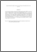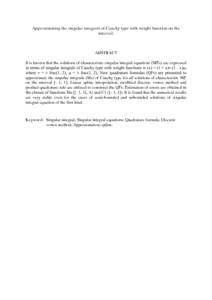# Approximating the singular integrals of Cauchy type with weight function on the interval.

## Citation

Eshkuratov, Zainidin K. and Nik Long, Nik Mohd Asri (2011) Approximating the singular integrals of Cauchy type with weight function on the interval. Journal of Computational and Applied Mathematics, 235 (16). pp. 4742-4753. ISSN 0377-0427

## Abstract

It is known that the solutions of characteristic singular integral equations (SIEs) are expressed in terms of singular integrals of Cauchy type with weight functions w (x) = (1 + x)ν (1 - x)μ, where ν = ± frac(1, 2), μ = ± frac(1, 2). New quadrature formulas (QFs) are presented to approximate the singular integrals (SIs) of Cauchy type for all solutions of characteristic SIE on the interval [- 1, 1]. Linear spline interpolation, modified discrete vortex method and product quadrature rule are utilized to construct the QFs. Estimation of errors are obtained in the classes of functions Hα ([- 1, 1], A) and C1 ([- 1, 1]). It is found that the numerical results are very stable even for the cases of semi-bounded and unbounded solutions of singular integral equation of the first kind.Preview
PDF (Abstract)
Approximating the singular integrals of Cauchy type with weight function on the interval.pdfView Item